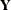# The HPPLS Procedure

### MODEL Statement

• MODEL response-variables = predictor-effects </ options>;

The MODEL statement names the responses and the predictors, which determine theandmatrices of the model, respectively. You can simply list the names of the predictor variables as the model effects, but you can also specify other types of effects, including polynomial effects and interactions. For information about constructing the model effects, see the section Specification and Parameterization of Model Effects, of Chapter 4: Shared Statistical Concepts.

The MODEL statement is required. You can specify only one MODEL statement.

You can specify the following options in the MODEL statement after a slash (/).

INTERCEPT

overrides the default, in which the responses and predictors are centered. When responses and predictors are centered, no intercept is required in the model.

SOLUTION

lists the coefficients of the final predictive model for the responses. The coefficients for predicting the centered and scaled responses that are based on the centered and scaled predictors are displayed, in addition to the coefficients for predicting the raw responses that are based on the raw predictors.